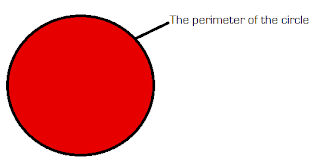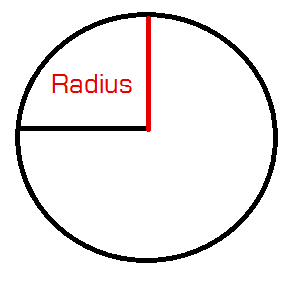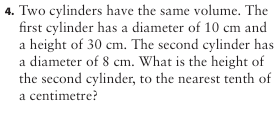## Tuesday, March 15, 2011

### Surface Area- cylinders.

Cylinders:
The bases of the cylinder are circles.

Circumference:
-The perimeter of a circle .Diameter:
- Halfs the circle.- Half of the diatmeter*Formulas :
Finding the circumference:
πd= c
Finding the diamer :

2r=d or c/π= d
d/2= r

*Area of circle:
π x r 2

* Homework questions :)A= πr2
A = 314 x 3.2 2
A= 3.14 x 10.24
A= 232.15 cm
2

A=
π r2
A= 3.14 x 6
2
A= 3.14 x 36
A=113.04cm
2

d/2= r
25/2 = r
25/2= 12.5
A=
πr2
A=3.14 x 6
2
A= 3.14 x 36
A= 490.526 cm
2

A=
πr2
A= 3.14 x 16.5
2
A- 3.14 x 272.25
A= 854.865 cm
2

c/ =d
18.84/ 3.14=d
18.84/ 3.14= 6
d/2= r
6/2= r
6/2= 3
A=
πr2
A= 3.14x 3
2
A= 3.14 x 9
A= 28.26 cm
2

c/ = d
31.4/ 3.14= d
31.4/3.14= 10
d/2 = r
10/2= r
10/2 = 5
A=
πr2
A= 3.14 x 5
2
A= 3.14 x 25
A = 78.5 cm
2

d/2 = r
15/2 = r
15/2= 7.5
A=
πr2
A= 3.14 x 7.5
2
A= 3.14 x 56.25
A= 176.63 cm2

Cylinder Volume and Volume Problemsa) d/2= r
10/2= 5cm^2

v= (πr^2) * h
v= (3.14 x 5 x 5 ) x 20
V= (78.5) x 20
V= 1570cm^3

b) d/2= r
1/2= o.5m^2

v= (πr^2) x h
v= (3.14 x 0.5 x o.5) x 1
v= (0.785) x 1
v= o.785m^3

c) d/2= r
18/2= 9cm^2

v= (πr^2) x h
v= (3.14 x 9 x 9) x 7.5
v= 254.34 x 7.5
v= 1907.55cm^31.2.